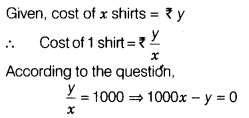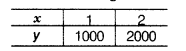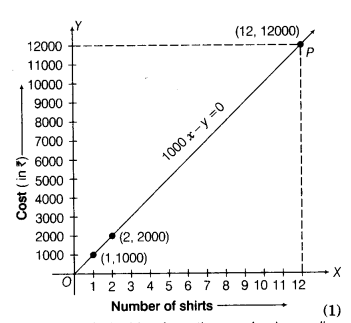# The cost of a shirt of a particular brand is ₹ 1000. Write a linear equation

The cost of a shirt of a particular brand is ₹ 1000. Write a linear equation, when the cost of x shirts is ₹ y. Draw the graph of this equation and find the cost of 12 such shirts from the graph.which is the required linear equation.
Eq. (i) can be written as y = 1000x.
When x = 1 then y = 1000 × 1 = 1000
When x =2, then y = 1000 × 2 = 2000
Thus, we have the following tableNow, plot the points (1,1000) and (2,2000) on the graph paper and join them by a line to get required graph.For the cost of 12 shirts from the graph, draw a line parallel to Y-axis from a point x = 12 on X-axis, which intersects the graph at point P.
Draw a perpendicular from point P on Y-axis, which intersects the Y-axis at y = 12000.
Thus, the cost of 12 shirts is ₹12000.